Altitude and Median of a triangle

Chapter 6 Class 7 Triangle and its Properties
Concept wise

Perpendicular from vertex to the opposite side of the triangle is the altitude of the triangle.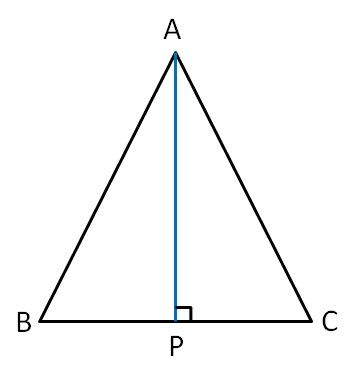Here, AP ⊥ BC

So, AP is the altitude of ∆ABC

We also sometimes call altitude as height of triangle.

Similarly, we can draw altitude from point B.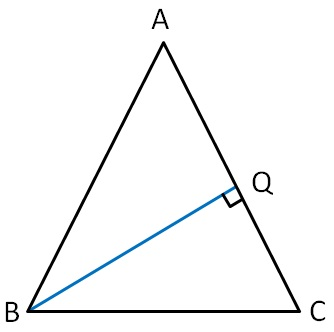Here, BQ ⊥ AC

So, BQ is the altitude of ∆ABC

Similarly, we can draw altitude from point C.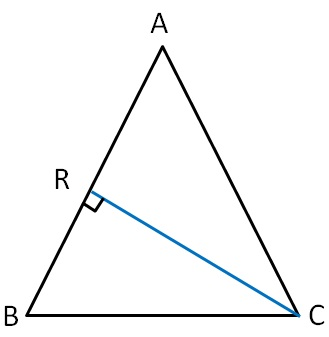Here, CR ⊥ AB

So, CR is the altitude of ∆ABC

So, altitudes of ∆ABC can be,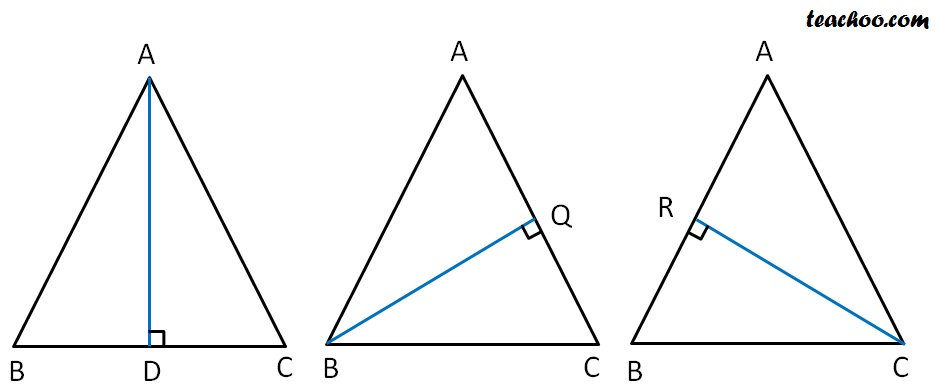## For an obtuse angled triangle ∆ABC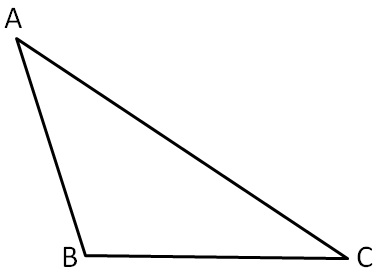Altitudes are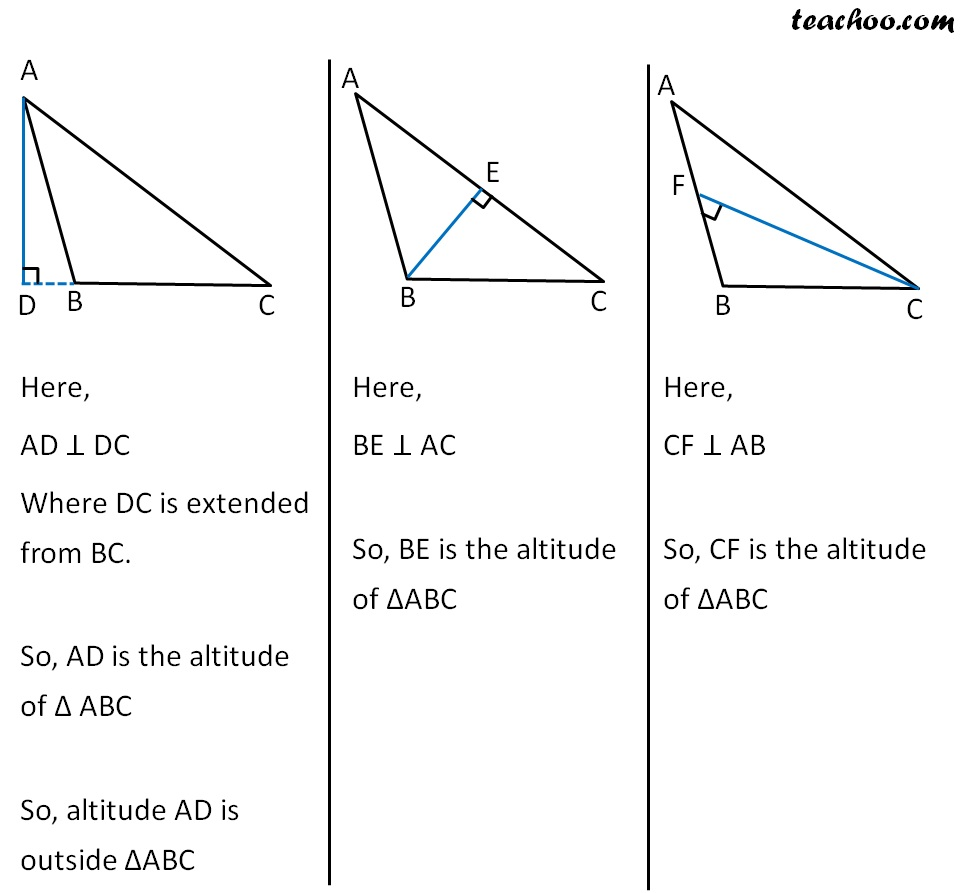Now,

In a right angled triangle. ∆ABC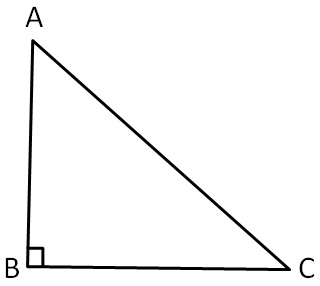Altitudes are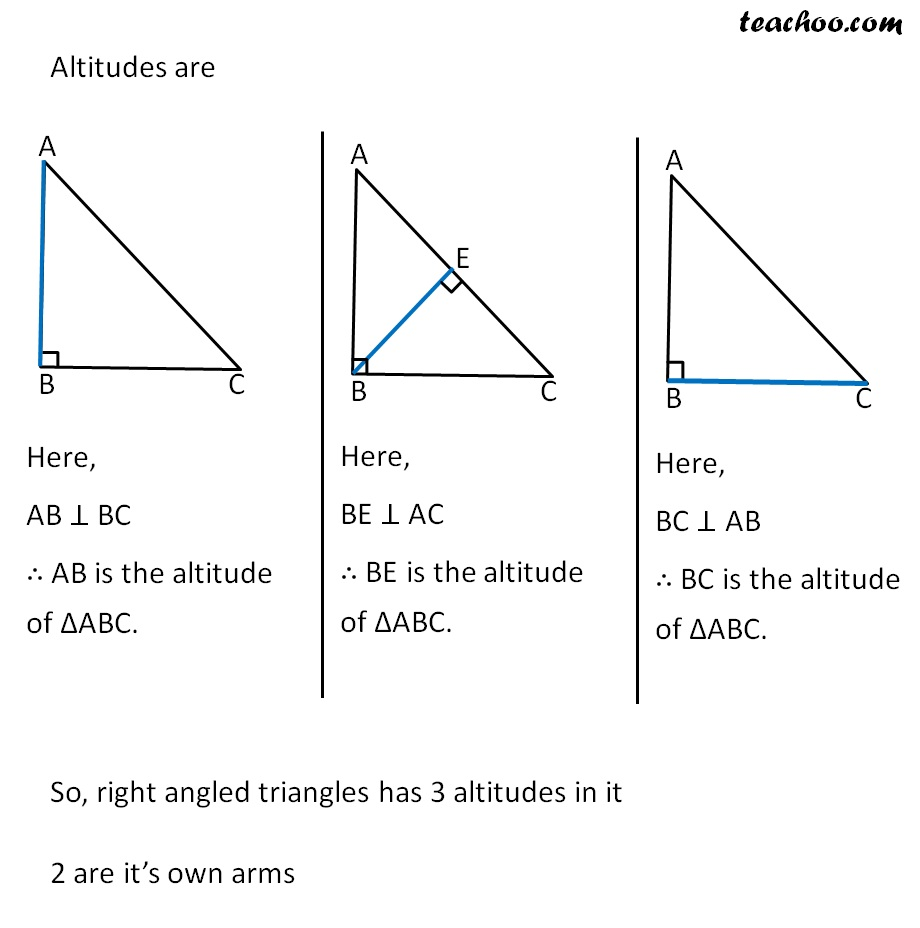So, right angled triangles has 3 altitudes in it

2 are it’s own arms

Learn in your speed, with individual attention - Teachoo Maths 1-on-1 Class